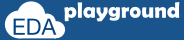# dist Constraint in SystemVerilog

Constraint provides control on randomization, from which the user can control the values on randomization. it would be good if it's possible to control the occurrence or repetition of the same value on randomization.yes its possible, with dist operator, some values can be allocated more often to a random variable. this is called a weighted distribution. dist is an operator, it takes a list of values and weights, separated by := or :/ operator.

## weighted distribution

As the name says, in weighted distribution weight will be specified to the values inside the constraint block. Value with the more weight will get allocated more often to a random variable.

### syntax

```value := weight   or
value :/ weight
```

Value   - desired value to a random variable
weight - indicates how often the value needs to be considered on randomization

• The values and weights can be constants or variables,
• value can be single or a range
• the default weight of an unspecified value is := 1
• the sum of weights need not be a 100

The := operator assigns the specified weight to the item, or if the item is a range, specified weight to every value in the range.

```addr dist { 2 := 5, [10:12] := 8 };

for addr == 2 , weight 5
```

The :/ operator assigns the specified weight to the item, or if the item is a range, specified weight/n to every value in the range. where n is the number of values in the range.

```addr dist { 2 :/ 5, [10:12] :/ 8 };

for addr == 2 , weight 5
```

### weighted distribution constraint examples

#### randomization with dist operator

In the example below,
On randomization, the possibility of 'addr' is getting the value of 10 is more than 7 and 2. this is because of weight specified to get value 10 is more than the other two values.

```class packet;

constraint addr_range { addr dist { 2 := 5, 7 := 8, 10 := 12 }; }
endclass

module constr_dist;
initial begin
packet pkt;
pkt = new();
```
```    \$display("------------------------------------");
repeat(10) begin
pkt.randomize();
end
\$display("------------------------------------");
end
endmodule```

Simulator Output
------------------------------------
------------------------------------
Execute the above code on#### difference between := and :/ dist operator

In the below example,

```class packet;

constraint addr_1_range {   addr_1 dist { 2 := 5, [10:12] := 8 }; }
constraint addr_2_range {   addr_2 dist { 2 :/ 5, [10:12] :/ 8 }; }
endclass

module constr_dist;
initial begin
packet pkt;
pkt = new();

\$display("------------------------------------");
repeat(10) begin
pkt.randomize();
end
\$display("------------------------------------");
```
```    \$display("------------------------------------");
repeat(10) begin
pkt.randomize();
end
\$display("------------------------------------");
end
endmodule```

Simulator Output
------------------------------------
------------------------------------
------------------------------------
Execute the above code on